# Haar condition

(diff) ← Older revision | Latest revision (diff) | Newer revision → (diff)

A condition on continuous functions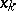,, that are linearly independent on a bounded closed setof a Euclidean space. The Haar condition, stated by A. Haar , ensures for any continuous functiononthe uniqueness of the polynomial of best approximation in the system, that is, of the polynomial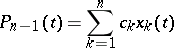(*)

for which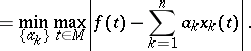The Haar condition says that any non-trivial polynomial of the form (*) can have at mostdistinct zeros on. For any continuous functiononthere exists a unique polynomial of best approximation in the system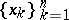if and only if the system satisfies the Haar condition. A system of functions satisfying the Haar condition is called a Chebyshev system. For such systems the Chebyshev theorem and the de la Vallée-Poussin theorem (on alternation) hold. The Haar condition is sufficient for the uniqueness of the polynomial of best approximation in the systemwith respect to the metric of(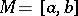) for any continuous function on.

How to Cite This Entry:
Haar condition. Encyclopedia of Mathematics. URL: http://encyclopediaofmath.org/index.php?title=Haar_condition&oldid=11643
This article was adapted from an original article by Yu.N. Subbotin (originator), which appeared in Encyclopedia of Mathematics - ISBN 1402006098. See original article